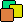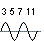Prime I.T. Help Prime I.T. :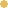Bitcoin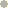Litecoin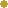DodgecoinVersion Française

# Trial division

Note : This article has been translated using an automatic translator and one pass was made to remove biggest errors.
If you want to contribute to the readability of this article, you can notify me translations errors (a word, a sentence, a paragraph) by contacting me at the email address at the bottom of the page. Thanks

## The principle

As its name suggests, the method "Trial Division" allows to find a factor of a given integer by trying to divide this number by all others are inferior.
This method is extremely simple (even simplistic) to find a factor of a number and does not require complex mathematical knowledge.
In fact this method repeat verbatim the definition of a prime as a prime number is a natural number that is divided by 1 and itself, prove that a number is prime thus requires checking that no number divides it and conversely a number is not prime (composite) if we find at least one factor.

Regarding the complexity, the algorithm is in the worst case O(n) or O(sqrt(n)) after optimization (we'll see further down). This complexity is not really great when we compare it with other existing algorithms but the method is interesting because it is relatively quick to find small factors of a number. It should also be noted that this algorithm can be used in 3 major problems on primes, namely: primality testing, factoring and finding a list of prime while the other algorithms are confined to their specialty (the Sieve of Eratosthenes can not factor a number...).

We'll explore this method to find all primes up to a limit N and compare times obtained with the Sieve of Eratosthenes and the Sieve of Atkin.
But we can start to think about the result will give the method Trial division and reflect it seems not so bad because we run through the numbers from 1 to N and we'll quickly find small factors of these numbers... And these numbers with small factors "there are many" for example if we take a random number, there is 1 chance in 2 that it is divisible by 2 (the smallest factor in integers), 1 chance in 3 to be divisible by 3, and so on...

## First implementation

From a computational point of view, so we need several things :

• An array of Boolean 2 to N as to whether a number is prime or not.
• A loop over the numbers from 1 to N which we will apply the test of Trial division.
• A second nested loop trying divisions with another number ranging from 1 to N.

Before launching on the implementation of this algorithm, we will start by making obvious optimizations :
- On the first loop, we avoid testing even numbers they are trivially not prime as divisible by 2. For this, the course of the first loop will be with a step of 2.
- On the 2nd optimization, it will be on the 2nd loop where we will test division only up to square root. Why ? Because if a number is not prime and therefore composite, it can be written in the form n = p * q and obviously one of the two factors p or q is less than the square root of n. Below, here are 2 very similar demos based on a reductio ad absurdum, proving that one of the two factors is less than the square root of n.

• Suppose p > √n and q > √n
Means that p * q > √n * √n but √n * √n = n
Thus p * q > n hence contradiction
• Suppose p > √n and q > √n
Means that there are two positive numbers x and y such as p = x + √n and q = y + √n
If we multiply p by q we have p * q = n = (x + √n) * (y + √n) = xy + √n(x * y) + n
ie : xy + √n(x * y) = 0
But all numbers of this equation are positive, we can not obtain 0 hence contradiction

private static final int MAX = 10000000;
private static boolean[] array = new boolean[MAX];
//--//
array[2true;

for (int i = 3; i < MAX; i += 2) {
sqrtN = Math.round(Math.sqrt(i)) 1;
premier = true;

for (int j = 3; j < sqrtN; j += 2) {
if ((i % j== 0) {
premier = false;
break;
}
}
array[i= premier;
}

MAXPi(MAX)Time (sec)
1 000 00078 4980.906
10 000 000664 57922.407
100 000 0005 761 455Too long !

So ! Let us not hide the results are very bad for the moment because in comparison with the sieve of Eratosthenes we have 0.906 seconds against 0.031 for the first non-optimized implementation, which is 30 times longer ! Worse, I stopped the test for 100 million because the cpu from my old AMD 3200+ 6 years of age ran a little too much for my taste...
Ok it is time to improve that.

## Second implementation

To improve the time it is absolutely necessary to succeed to make less division than the first implementation and for that we go from one observation :
Suppose we want to test the Trial division of the number 105 (3*5*7) we see that "i%j==0" will return true (because divisible) for 3, for 5, for 7 but also for 15, for 21 and for 35.
Invert the argument, take the number 437 (19*23). We see that this number is not divisible by 3 or by 5 and will not by 15 (3*5).
Our first implementation of the Trail division shows that we are doing too munch divisions as we try to divide a number X with composite numbers whose prime components are not divisible with this number X. The ideal to solve this problem would be to do successive divisions with only prime numbers...
We are going around in circles because the program is precisely calculate these primes but in fact it is possible (!) Since we are currently calculate them, we will use those already discovered to find the following. It is a bit like reinjection of a result to find the final result.

Finally we note that the array does not contain no more boolean but primes themselves. This means that to fix the size of this array, we need to know the number of prime numbers when we do a test on a value of N and for that there is the prime number theorem saying that Pi(N) approaches N / ln(N) when N tends to infinity (Wikipedia: Prime number theorem). Here our tests are in the order of hundreds of millions, we are very far from infinity :) and so we are obliged to fix a small delta (1.1).

private static final int MAX = 10000000;
private static final int ARRAY_SIZE = (intMath.round((MAX * 1.1/ Math.log(MAX));
private static int[] array = new int[ARRAY_SIZE];
//--//
int pos = 0;
array[pos++2;

for (int i = 3; i < MAX; i += 2) {
sqrtN = Math.round(Math.sqrt(i)) 1;

for (int j = 0; j < pos; j++) {
int value = array[j];
if (value > sqrtN) {
premier = true;
break;
}
if ((i % value== 0) {
premier = false;
break;
}
}

if (premier) {
array[pos++= i;
}
}

MAXPi(MAX)Time (sec)
1 000 00078 4980.453
10 000 000664 5798.734
100 000 0005 761 455188.172

Indeed we won a lot of time with this optimization! But we are still far from the Sieve of Eratosthenes... Try to do better. source

## Third implementation

To optimize the algorithm, we are going to have the same approach for the optimization of the Sieve of Atkin, we will adress one of the operators which is an expensive operation in computing time. Here it will be the square root.

If we look at the values that take the integer part of the square root we see that they follow the sequence : 3 3  4 4 4  5 5 5 5  6 6 6 6 6... and we can deduce the rule "the integer n representing the integer part of the square root is repeated n-1 times".
You can find more information on Wikipedia, unfortunately i did not find the English version of the following address : Racine carrée / rubrique "Les racines carrées, approximations entières".

private static final int MAX = 100000000;
private static final int ARRAY_SIZE = (intMath.round((MAX * 1.1/ Math.log(MAX));
private static int[] array = new int[ARRAY_SIZE];
//--//
int pos = 0;
array[pos++2;

int sqrtN = 3;
int countSqrtN = 2;

for (int i = 3; i < MAX; i += 2) {
for (int j = 0; j < pos; j++) {
int value = array[j];
if (value > sqrtN) {
premier = true;
break;
}
if ((i % value== 0) {
premier = false;
break;
}
}

if (premier) {
array[pos++= i;
}

if (--countSqrtN == 0) {
countSqrtN = sqrtN;
sqrtN++;
}
}

MAXPi(MAX)Time (sec)
1 000 00078 4980.344
10 000 000664 5797.078
100 000 0005 761 455155.25

Gradually we improve the algorithm but this time we reach at the limit of optimization. source

## In conclusion

So we see that we are very far by using the Trial division compared to others sieves and despite 3 optimizations we have a ratio of 1 to 11 with a non-optimized Sieve of Eratosthenes. We do not even tickled the sieves...
The difference lies in the philosophy of the algorithm, it tries to determine the primality in a timely manner (I choose this number is it prime or not ?) and we must run all the numbers from 1 to N in order to start the divisions. In comparison a sieve takes all the numbers to apply "a sieve function" above and in general this function is sufficient if it is applied up to square root of N.

Against by this method has some advantages, as explained at the beginning of the article it is easy to quickly find small factors, it can be used for testing primality, factoring and finding a list prime. Moreover, on this last point, the algorithm is flexible about the limits of research because we do not have to start with 1 unlike the Sieve of Eratosthenes and Atkin.

A website created by Les composants associés (cpas)